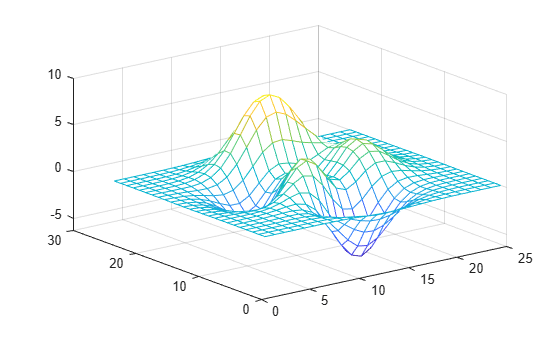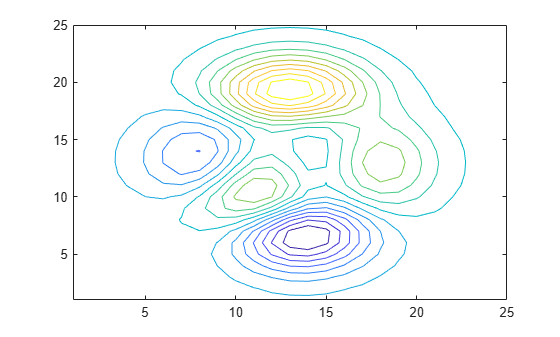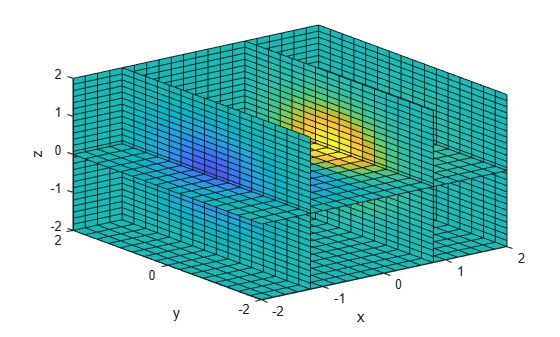# Creating 3-D Plots

This example shows how to create a variety of 3-D plots in MATLAB®.

### Mesh Plot

The `mesh` function creates a wireframe mesh. By default, the color of the mesh is proportional to the surface height.

```z = peaks(25); figure mesh(z)```### Surface Plot

The `surf` function is used to create a 3-D surface plot.

`surf(z)`The `surfl` function creates a surface plot with colormap-based lighting. For smoother color transitions, use a colormap with linear intensity variation such as `pink`.

```surfl(z) colormap(pink) % change color map shading interp % interpolate colors across lines and faces```### Contour Plot

The `contour` function is used to create a plot with contour lines of constant value.

```contour(z,16) colormap default % change color map```### Quiver Plot

The `quiver` function plots 2-D vectors as arrows.

```x = -2:.2:2; y = -1:.2:1; [xx,yy] = meshgrid(x,y); zz = xx.*exp(-xx.^2-yy.^2); [px,py] = gradient(zz,.2,.2); quiver(x,y,px,py) xlim([-2.5 2.5]) % set limits of x axis```### Slices through 3-D Volumes

The `slice` function displays data at planes that slice through volumetric data.

```x = -2:.2:2; y = -2:.25:2; z = -2:.16:2; [x,y,z] = meshgrid(x,y,z); v = x.*exp(-x.^2-y.^2-z.^2); xslice = [-1.2,.8,2]; % location of y-z planes yslice = 2; % location of x-z plane zslice = [-2,0]; % location of x-y planes slice(x,y,z,v,xslice,yslice,zslice) xlabel('x') ylabel('y') zlabel('z')```